StatLect

# Non-homogeneous system

A non-homogeneous system of equations is a system in which the vector of constants on the right-hand side of the equals sign is non-zero.

This lecture presents a general characterization of the solutions of a non-homogeneous system.

We recommend to read the lecture on homogeneous systems before reading this one.## Definition

A non-homogenous system has the formwhereis amatrix of coefficients,is avector of unknowns andis anon-zero vector of constants.

Example Consider the systemIts matrix form isThe system is non-homogeneous because the vector of constants is## Equivalent system in row echelon form

By means of elementary row operations, a non-homogenous system can be transformed into an equivalent systemwhere the coefficient matrixis in row echelon form (REF).

The equivalent system in row echelon form has the same solutions as the original one.

## Existence of solutions

Unlike homogeneous systems, that are guaranteed to always have at least one solution (the so-called trivial solution), non-homogeneous systems may not have a solution.

As demonstrated in the lecture on row echelon forms, if the REF matrixhas a zero rowand, at the same time,, then the system has no solution. If there are no such rows, then the system has at least one solution.

## Basic and non-basic variables

The columns of an REF matrix are of two kinds:

• basic columns: they contain a pivot (i.e., a non-zero entry such that all the other entries below it and to its left are equal to zero);

• non-basic columns: they do not contain a pivot.

Here is a simple example.

Example Consider theREF matrixThe first and the third columns are basic, while the second one is non-basic.

## Particular solution

When the system has a solution, we can assign arbitrarily chosen values to the non-basic variables and then use the back-substitution algorithm to find the values of the basic variables that solve the system. The solution thus found, for a specific choice of the non-basic variables, is called a particular solution of the system.

Example Consider the systemwhereis given in the previous example and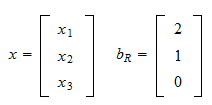Since the third row ofis zero, we need to check the third entry of. It is zero, so the system has a solution. The variableis non-basic and we can assign an arbitrary value to it. We choose. We can now start the back-substitution algorithm. The second equation isand the first equation isorThus, a particular solution of the system is## Associated homogeneous system

A general solution of a system is a characterization of the set of all its possible solutions.

The general solution of a non-homogeneous system is usually provided by relating it to the general solution of its associated homogeneous system.

Letbe a non-homogeneous system. Then, its associated homogeneous system is## General solution

The general solution of a non-homogeneous system is characterized as follows.

Proposition Letbe any particular solution of (1). Then,is a solution of (1) if and only ifis a solution of (2).

Proof

The hypothesis is thatIf (1) holds, then we can subtract (3) from (1) to obtainConversely, if (4) holds, then we can add (3) to it, so as to obtain (1).

In other words, we can derive the set of all the solutions of the non-homogeneous system (1) in three steps:

• we derive the set of solutions of the homogeneous system (2):• we derive a specific solutionof (1);

• we obtain the general solution of the non-homogeneous system asHere is an example.

Example Consider the following non-homogeneous system:where the coefficient matrix is already in row echelon form:and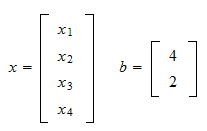There are no zero rows, so the system is guaranteed to have a solution. The first two columns are basic, while the last two are non-basic. We can find a particular solution by setting the non-basic variables to zero (). After doing so, the solution becomes readable from the matrix of coefficients:,. Thus, we have a particular solutionAlso the solution of the associated homogeneous system is immediate to find: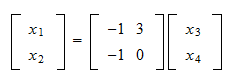Therefore, the solution of the given non-homogeneous system is the set of all vectorsthat satisfy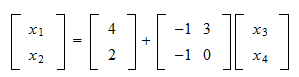## Solved exercises

Below you can find some exercises with explained solutions.

### Exercise 1

Find the general solution of the systemwhereandSolution

To facilitate things, we are going to transform the system into an equivalent one in reduced row echelon form. We divide the second equation by; then, we subtract two times the second equation from the first one. The result is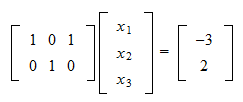The existence of a solution is guaranteed by the fact that there are no zero rows in the reduced echelon form. We can derive a particular solution by setting(sinceis non-basic). As a consequence, the particular solution isThe general solution of the associated homogeneous system is the set of all vectorsthat satisfyThe general solution of the given non-homogeneous system contains all vectors that satisfy